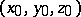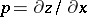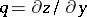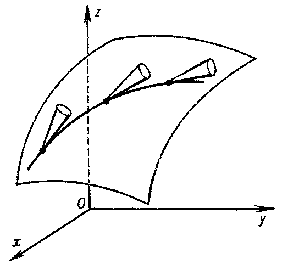# Monge cone

(diff) ← Older revision | Latest revision (diff) | Newer revision → (diff)

directing cone

The envelope of the tangent planes to the integral surface at a pointof a partial differential equation(*)

where,. Ifis a non-linear function inand, then the general case holds: The tangent planes form a one-parameter family of planes passing through a fixed point; their envelope is a cone. Ifis a linear function inand, then a bundle of planes passing through a line is obtained, that is, the Monge cone degenerates to the so-called Monge axis. The directions of the generators of the Monge cone corresponding to some pointare called characteristic directions. A line on the integral surface which is tangent at each point to a corresponding generator of the Monge cone is called a characteristic line, a characteristic, a focal curve, or a Monge curve.Figure: m064630a

The geometric interpretation (see Fig.) of equation (*), as a field of directing cones, was given by G. Monge (1807).